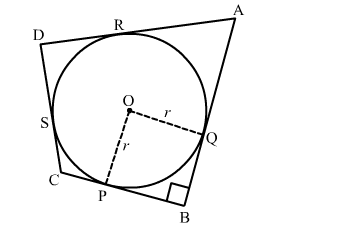# In the given figure, a circle with centre O, is inscribed in a quadrilateral ABCD such that it touches the side BC,`
Question:

In the given figure, a circle with centre O, is inscribed in a quadrilateral ABCD such that it touches the side BC, AB, AD and CD at points P, Q, R and S respectively. If AB = 29 cm, AD = 23 cm, ∠B = 90 and DS = 5 cm then find the radius of the circle.Solution:

We know that tangent segments to a circle from the same external point are congruent.
Now, we have
DS = DR, AR = AQ
⇒ AR + RD = 23
⇒ AR = 23 − RD
⇒ AR = 23 − 5          [∵ DS = DR = 5]
⇒ AR = 18 cm
Again, AB = 29 cm
⇒ AQ + QB = 29
⇒ QB = 29 − AQ
⇒ QB = 29 − 18          [∵ AR = AQ = 18]
⇒ QB = 11 cm
Since all the angles are in a quadrilateral BQOP are right angles and OP = BQ.
Hence, BQOP is a square.
We know that all the sides of square are equal.
Therefore, BQ = PO = 11 cm
Hence, the radius of the circle is 11 cm.